### 5.3Implementation of the Improved Implication Architecture

#### 5.3.1Structural Asymmetry

By replacing the MTJ devices with 1T/1MTJ cells, the implication logic gates are realized in MRAM arrays to provide large-scale non-volatile magnetic circuits (Fig. 5.3). Due to the structural asymmetry caused by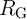, two MRAM arrays are required in this asymmetric implementation. For performing the implication operation, a source (target) MTJ can be selected only in the source (target) MRAM array which is (not) serially connected to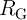. Although this architecture enables independent STT writing of the input MTJs to eliminate the difference between reading, writing, and performing logic operations, intermediate read/write operations are required to readout the output of any logic operation from the target array and to write it in the source array as an input for the next logic steps. In fact, as there is a need for a physical resistor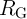which is connected in series to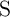,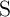(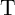) can be used only as source (target) MTJ for the implication operations and the logic result stored in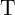cannot be used as a source input for the next implication operation. Therefore, performing implication operation between two inputs from the same array is not possible without intermediate read/write operations.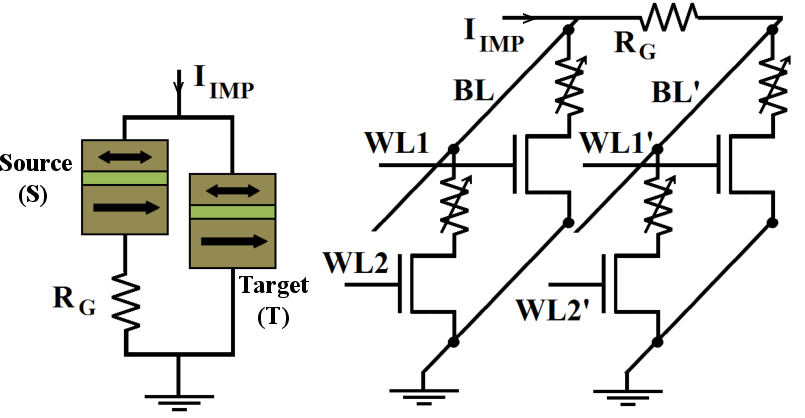Figure 5.3.: Asymmetric 1T/1MTJ-based implementation (right) of the CC-IMP logic gate (left).

Fig. 5.4 shows a simplified implication logic circuit architecture based on the STT-MRAM architecture to realize the MTJ-based current-controlled implication gate (Fig. 5.4). This circuit enables stateful logic for which the need of using extra charge-based logic gates is eliminated and the memory cells serve simultaneously as logic gates and latches via implication operation. The implication operation between two cells C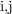and C(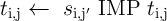) can be performed by simultaneous selection of the-th WL, the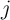-th (target) and the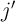-th (source) SLs which are connected to the ground directly and via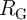, respectively, and by applying the current source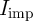to the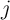-th and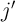-th BLs. The result of the implication operation is written in C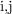.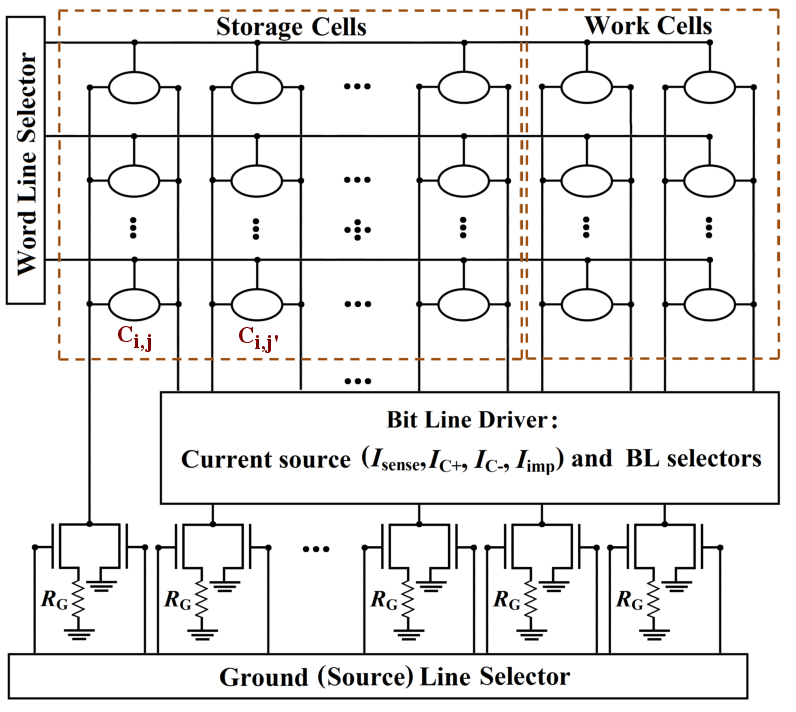Figure 5.4.: Asymmetric MRAM-based implication logic architecture.

Compared to the common STT-MRAM architecture, the SL and the BL drivers are more complicated as they have to provide more selection capabilities. Furthermore, two work cells are added to each WL, since it has been shown that with two additional memory elements all Boolean functions can be performed on any number of the storage cells . These work cells can also be used to connect different WLs. Indeed, in order to perform the implication between memory cells from different WLs, one has to copy the logic data stored in a memory cell into a work cell from the other WL. This increases the required time and energy consumption and limits the flexibility of the computation.

It should be noted that the nonzero ON resistance of the access transistors (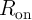) decreases the effective TMR ratio of the 1T/1MTJ cells which can be defined as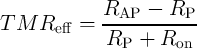(5.5)

Therefore, a robust implication operation needs MTJs with sufficiently high TMR ratio and electrical resistance. According to Fig. 4.17b, an implication reliability of 99.9% requires a TMR ratio higher than 250% when the effective TMR ratio of a 1T/1MTJ is decreased by about 10%-30% based on the MTJ and the transistor devices characterized in .

#### 5.3.2Addressing the Asymmetry Issue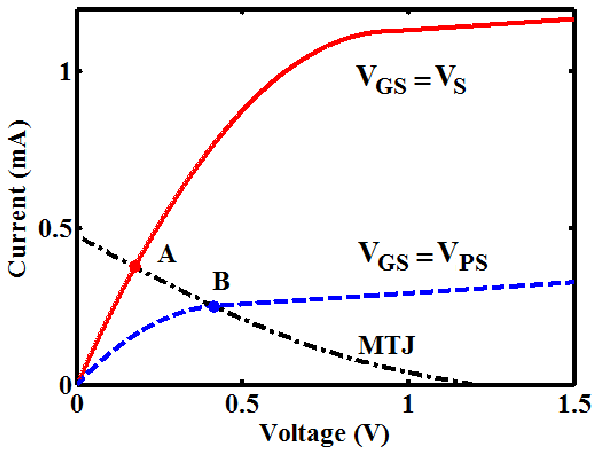Figure 5.5.: Bias points of the access transistor in a 1T/1MTJ cell for the selecting (point A) and pre-selecting (point B) voltages applied to the word line of the cell.

The inherent asymmetry of the proposed implication logic gate causes a significant limitation in the flexibility of the computations and forces extra read/write operations in the MRAM-based architectures shown in Fig. 5.3 and Fig. 5.4 as discussed before. This problems can be addressed by an innovative solution for the asymmetry issue by using the access transistors as voltage-controlled resistors to eliminate the need for a physical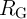. If voltage pulses with different amplitudes are applied to the different WLs, the transistors have different bias points (Fig. 5.5) and thus exhibit different channel resistances. Fig. 5.6a shows the MTJ- and the MRAM-based CC-IMP circuit topologies. In the MRAM array, the structural asymmetry required for the CC-IMP is provided, when the select and pre-select voltage signals (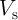and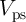) are applied to two arbitrary WLs. As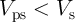, the transistors exhibit different channel resistances and the required structural asymmetry is implicitly provided by the pre-selected transistor featuring a higher resistance which acts as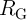. The logic operation is performed by applying simultaneously the current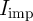to the common BL and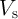and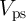to the WLs of the target and the source 1T/1MTJ cells, respectively. The logic result is stored as the final resistance state of the selected (target) MTJ, which can be used now as a source input by pre-selection in the next operations. This significantly reduces the complexity, energy consumption, and delay as it eliminates the need for extra hardware like the source line selector shown required in the MRAM architecture (shown Fig. 5.4) as well as the intermediate read/write operations needed for reading (writing) the target (source) data of the current (next) logic stage.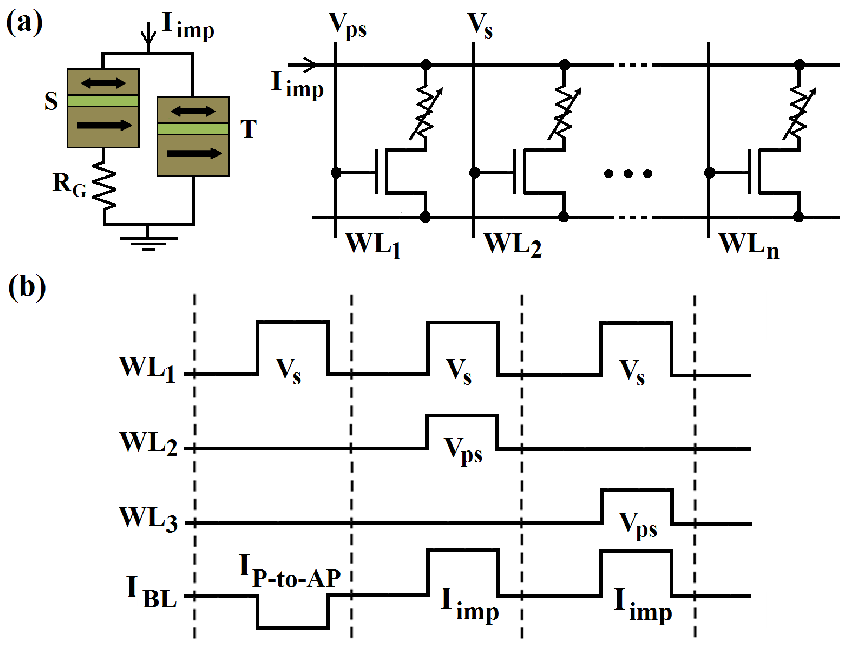Figure 5.6.: (a) MTJ- and MRAM-based implication logic architectures with no need for a physical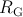. (b) Circuit signals for performing the universal NOR operation in MRAM-based implication logic architecture.

Fig. 5.6b shows the required circuit signals to implement the universal NOR operation (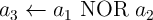) in three steps, one TRUE and two NIMP operations, as shown in Eq. 3.2. According to Eq. 4.27, the reliability of the implication-based NOR is then obtained as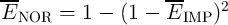which is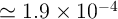for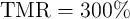. For a given MTJ and transistor device characteristics, the values of the circuit parameter (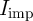and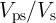) are optimized (Fig. 5.7) by using Eq. 5.1 and the reliability model presented in the previous chapter.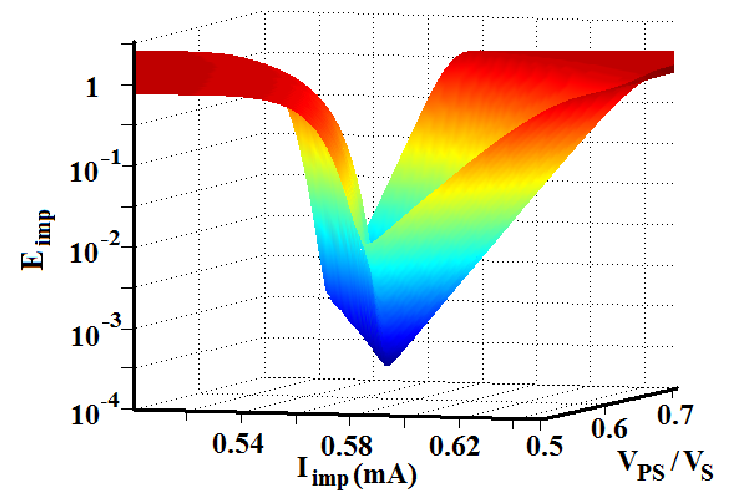Figure 5.7.: Circuit parameters optimization for minimum error probability of the symmetric implication gate.